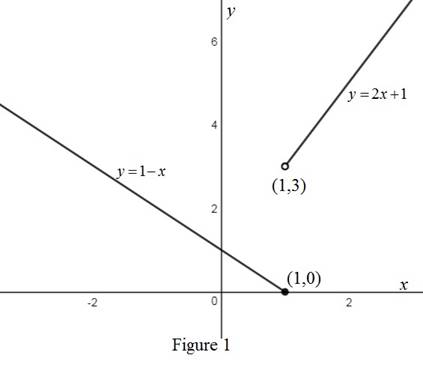# The value of f ( − 2 ) and f ( 1 ) for the function f ( x ) = { 1 − x if x ≤ 1 2 x + 1 if x &gt; 1### Precalculus: Mathematics for Calcu...

6th Edition
Stewart + 5 others
Publisher: Cengage Learning
ISBN: 9780840068071### Precalculus: Mathematics for Calcu...

6th Edition
Stewart + 5 others
Publisher: Cengage Learning
ISBN: 9780840068071

#### Solutions

Chapter 2, Problem 8T

(a)

To determine

## To find: The value of f(−2) and f(1) for the function f(x)={1−x        if x≤12x+1      if x>1

Expert Solution

The value of f(2) is 3 and f(1) is 0.

### Explanation of Solution

Given:

The piecewise defined function is, f(x)={1xifx12x+1ifx>1

Calculation:

The given function is piecewise defined function

So, for x1 function is defined as (1x) and for x>1 function is defined as (2x+1).

To calculate f(2) substitute x=2 in (1x) because 2 comes in domain of this function.

f(2)=1(2)=1+2=3

Thus, the value of f(2) is f(2)=3.

To calculate f(1), substitute x=1 in (1x) because 1 is included in the domain of (1x).

f(1)=11=0

Thus, the value of f(2) is 3 and f(1) is 0.

(b)

To determine

Expert Solution

### Explanation of Solution

The behavior of piecewise defined function is different for different domains.

For x1 the function is defined as (1x)

For x>1 the function is defined as (2x+1)

Sketch the graph of (1x) and (2x+1) using transformation.

Sketch the graph of y=x, shift it horizontally right by 1 unit then takes its reflection in x-axis to get the graph of y=1x.

Sketch the graph of y=x, stretch it vertically by factor of 2 and then shift it vertically up by 1 unit to get the graph of y=2x+1.

Sketch the piecewise defined function as shown in the figure below.From the above figure, it can be observed that there is a solid dot at (1,0) to show this point is included in the graph and there is open circle at (1,3) to show that this point is not included in the graph.

### Have a homework question?

Subscribe to bartleby learn! Ask subject matter experts 30 homework questions each month. Plus, you’ll have access to millions of step-by-step textbook answers!